# Equations and inequations with square root

#### Loki123

##### Junior Member
I have posted a few problems that gave me the same issue. Unfortunately, I haven't been able to understand the reasoning on why they are done the way they are. So I decided to sum up all my problems regarding that issue here in hopes to understand it.
The following picture includes two equations and one inequality. They are not solved completely; rather they all just state a condition revolving the square root.
1st and 3rd problem are equations and they both state that the RHS cannot be negative, that is it must be non-negative. I understand this because LHS is a square root and by definition the square root is always non-negative, that is equal or above 0.
The 2nd problem is giving me a headache. It is an inequality and it is solved by separating the problem into two options; one where x <= 0 and one where x>0. Why is this? My assumption is that although the LHS is always non-negative, the RHS in this case can be negative because it is an inequality. So If RHS is negative and since LHS is always non-negative, the inequality would work because LHS would be always greater than RHS. I tried to present this in a graph. Basically if -x is on the left side of 0, the square root is always greater, however, if -x is on the right side, in some cases the square root would not be greater.
Is this thinking correct? Even if it is, I am still a little bit confused, because why do we have to separate it into two options, why won't solving it like it is just do the job? If we combine x<=0 and x>0, x is basically all numbers.
Thank you,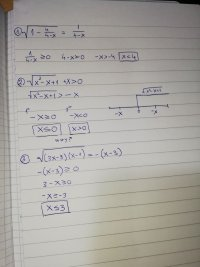#### Dr.Peterson

##### Elite Member
The 2nd problem is giving me a headache. It is an inequality and it is solved by separating the problem into two options; one where x <= 0 and one where x>0. Why is this? My assumption is that although the LHS is always non-negative, the RHS in this case can be negative because it is an inequality. So If RHS is negative and since LHS is always non-negative, the inequality would work because LHS would be always greater than RHS. I tried to present this in a graph. Basically if -x is on the left side of 0, the square root is always greater, however, if -x is on the right side, in some cases the square root would not be greater.
Specifically, the RHS can be negative because it is required to be LESS THAN a positive number, which is true of any negative number. Being less than a positive number does not make a number positive.

I am still a little bit confused, because why do we have to separate it into two options, why won't solving it like it is just do the job? If we combine x<=0 and x>0, x is basically all numbers.
It's misleading to ask "why do we HAVE TO"; there are often multiple ways to solve a given problem. Rather, your question is, "why do they choose to".

Now, I wish you had responded to what was said in the other thread, particularly my (implied) question about what was done next. If you had shown the entire answer, I could refer to that to show the need for two cases, in light of what they did. When you have a question about what someone did, you need to show all of what they did, and not just a little part.

But as I said there, I assume that the next step is to square both sides.

In that case, the reason for making separate cases is that squaring has different effects on positive and negative numbers. Consider this: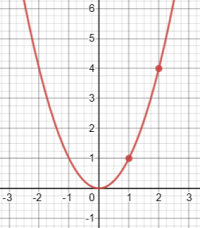For [imath]x>0[/imath], [imath]y = x^2[/imath] is an increasing function, so that given that [imath]2 > 1[/imath], we can conclude that [imath]2^2 > 1^2[/imath].

But if [imath]x<0[/imath], all bets are off: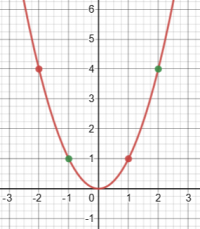Given that [imath]2 > -1[/imath], it is true that [imath]2^2 > (-1)^2[/imath] (the green points); but given that [imath]1 > -2[/imath], it is NOT true that [imath]1^2 > (-2)^2[/imath] (the red points). So if we don't require that the given values are both positive, we can't square them and expect the inequality to be preserved.

So we need to consider separate cases because we are going to be squaring.

So, assuming that the next step is to square and get [imath]x^2-x+1 > x^2[/imath], as I expect, then take an example: for [imath]x = 2[/imath], the original inequality is true, [imath]\sqrt{x^2-x+1}=\sqrt{2^2-2+1}=\sqrt{3}[/imath] while [imath]-x=-2[/imath], and [imath]\sqrt{3}> -2[/imath]; but the squared inequality is not: [imath]\sqrt{x^2-x+1}^2=x^2-x+1=2^2-2+1=3[/imath] while [imath](-x)^2=(-2)^2=4[/imath], and [imath]3 \not{>} 4[/imath].

So you can't square an inequality without imposing a restriction.

•Loki123

#### Dr.Peterson

##### Elite Member
Could you explain why they used different conditions than me?
You stated the cases, but don't seem to have done different things in each (which suggests that you don't really understand the reasoning fully):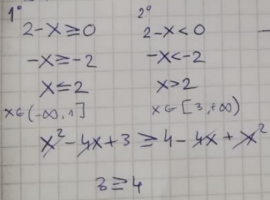It's only in the first case that you can square both sides of the inequality and conclude, as you show, that 3>=4.

In the second case, as I've said, you can't do that, but instead conclude that the inequality is always true, because the LHS is positive and the RHS is negative and therefore necessarily less than the LHS.

It's also worth observing that for this problem, unlike your initial question, the cases they use are not based on the sign of the RHS, but are just the two parts of the domain, each of which happens to imply the sign of the RHS. That aspect of their work is equivalent to what you did. The only difference is that in their first case, they didn't square, but just stated that the inequality is necessarily true.

•Loki123

#### Loki123

##### Junior Member
Thank you so much! I think I understand.

#### Dr.Peterson

##### Elite Member
Would you mind checking this one out? i got the correct answer, but I am not so sure about how I did it...

First: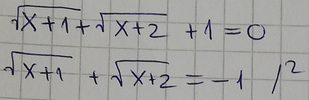You don't need to do the work that follows this, since clearly the LHS can't be negative. But that work isn't wrong, since you know both radicals are defined when [imath]x\ge 1[/imath]. On the other hand, I would have isolated a radical before squaring, to keep things simple. And you could again have stopped when you got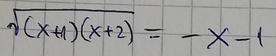since if [imath]x\ge 1[/imath], the RHS has to be negative.

Second: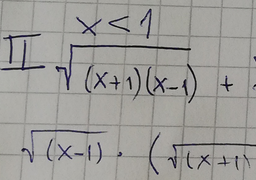Here you have stopped thinking. If you are assuming [imath]x<1[/imath], how can you write the square root of [imath]x-1[/imath], which is negative?

The whole point of using cases is to avoid that! In this case, I would simplify that first term as [imath]\sqrt{(-x-1)(-1+x)}=\sqrt{-x-1}\sqrt{-1+x}[/imath], where the second radical is defined. When is the first radical defined? You'll need to state that, too.

Since the second case is nonsense, you solved the problem by accident. That's not hard to do in a problem like this. But it won't get you a good grade, and doesn't teach you how to think carefully.

#### Loki123

##### Junior Member
I would simplify that first term as (−x−1)(−1+x)=−x−1−1+x\sqrt{(-x-1)(-1+x)}=\sqrt{-x-1}\sqrt{-1+x}(−x−1)(−1+x)=−x−1−1+x
I got lost here. Why rewrite it like that and how?

#### Loki123

##### Junior Member

First:
View attachment 29313
You don't need to do the work that follows this, since clearly the LHS can't be negative. But that work isn't wrong, since you know both radicals are defined when [imath]x\ge 1[/imath]. On the other hand, I would have isolated a radical before squaring, to keep things simple. And you could again have stopped when you got
View attachment 29314
since if [imath]x\ge 1[/imath], the RHS has to be negative.

Second:
View attachment 29315
Here you have stopped thinking. If you are assuming [imath]x<1[/imath], how can you write the square root of [imath]x-1[/imath], which is negative?

The whole point of using cases is to avoid that! In this case, I would simplify that first term as [imath]\sqrt{(-x-1)(-1+x)}=\sqrt{-x-1}\sqrt{-1+x}[/imath], where the second radical is defined. When is the first radical defined? You'll need to state that, too.

Since the second case is nonsense, you solved the problem by accident. That's not hard to do in a problem like this. But it won't get you a good grade, and doesn't teach you how to think carefully.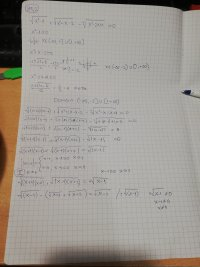Tried a different approach and got that x can't be one... Where did i go wrong??

#### Loki123

##### Junior Member

First:
View attachment 29313
You don't need to do the work that follows this, since clearly the LHS can't be negative. But that work isn't wrong, since you know both radicals are defined when [imath]x\ge 1[/imath]. On the other hand, I would have isolated a radical before squaring, to keep things simple. And you could again have stopped when you got
View attachment 29314
since if [imath]x\ge 1[/imath], the RHS has to be negative.

Second:
View attachment 29315
Here you have stopped thinking. If you are assuming [imath]x<1[/imath], how can you write the square root of [imath]x-1[/imath], which is negative?

The whole point of using cases is to avoid that! In this case, I would simplify that first term as [imath]\sqrt{(-x-1)(-1+x)}=\sqrt{-x-1}\sqrt{-1+x}[/imath], where the second radical is defined. When is the first radical defined? You'll need to state that, too.

Since the second case is nonsense, you solved the problem by accident. That's not hard to do in a problem like this. But it won't get you a good grade, and doesn't teach you how to think carefully.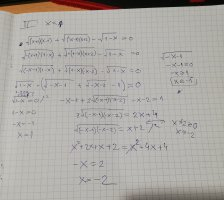I tried to attempt what you described, however, I am pretty sure I did the same thing as before...

#### Dr.Peterson

##### Elite Member
Tried a different approach and got that x can't be one... Where did i go wrong??
That doesn't imply that x is not 1; it assumes it in order to divide by 0! The equation you have there is in fact true if x=1.

I'm not convinced that your method is worth pursuing; but if you still want to, you need also to notice the domain of [imath]\sqrt{-x-2}[/imath]

Which of #7 and #10 shows the correct problem?

#### Loki123

##### Junior Member
That doesn't imply that x is not 1; it assumes it in order to divide by 0! The equation you have there is in fact true if x=1.

I'm not convinced that your method is worth pursuing; but if you still want to, you need also to notice the domain of [imath]\sqrt{-x-2}[/imath]

Which of #7 and #10 shows the correct problem?
Both. #7 is the entire problem. #10 is a different approach.

#### Dr.Peterson

##### Elite Member
Both. #7 is the entire problem. #10 is a different approach.
No, I'm not talking about the work, but about the equation itself. One has a + where the other has a -. I presume the former is a typo, as that equation has no solution.

Is the method you are using your own invention, or is it what you have been taught? I would use an entirely different method.

#### Loki123

##### Junior Member
No, I'm not talking about the work, but about the equation itself. One has a + where the other has a -. I presume the former is a typo, as that equation has no solution.

Is the method you are using your own invention, or is it what you have been taught? I would use an entirely different method.
It is -, I don't know how I got that wrong...